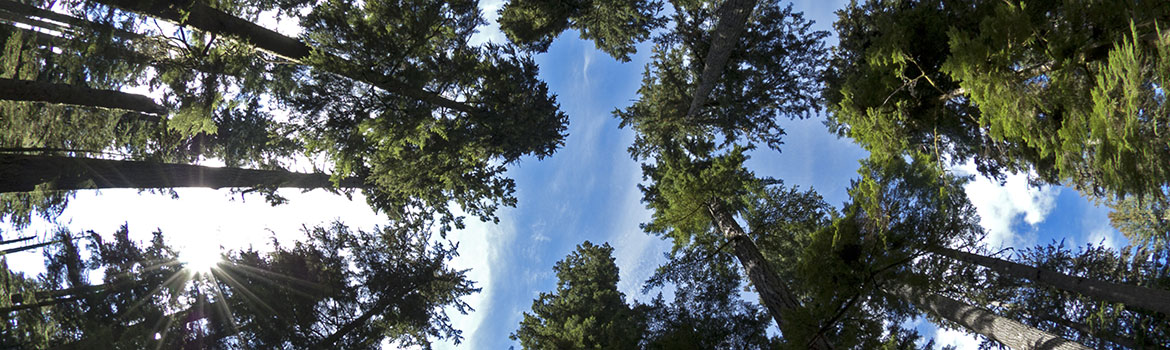# Model DescriptionThe 3PG(S) model

The 3-PG model (Landsberg and Waring 1997) is based on established biophysical relationships and constants.

3-PG(S) is a monthly time step model requiring average incoming photosynthetically active radiation (fp.a), mean daytime vapour pressure deficits (D), temperature extremes, monthly precipitation and estimates of soil water storage capacity and fertility.

The utilised portion of Øp.a (Øp.a.u) is obtained by reducing Øp.a by an amount determined by a series of modifiers derived from constraints imposed by:

• stomatal closure, associated with high day-time D;
• soil water, the difference between rainfall + available soil water and transpiration, calculated using the Penman-Monteith equation with canopy conductance modified by Leaf Area Index (L) and constrained by monthly estimates of D (reaching a maximum value of 0.02 ms-1).
• the effects of sub-freezing temperatures

Gross primary production (PG) is Øp.a. multiplied by a canopy quantum efficiency coefficient (ac).

3-PG(S) assumes total net primary production (PN) in temperate forests is a fixed fraction (0.47 ± 0.04) of PG

3-PG(S) partitions PN into below (PB) and above-ground biomass (PA), the latter in 3-PG into two (PS) and foliage (PF).

The fraction of PN allocated to root growth increases from 0.2 to 0.6 as the ratio Øp.a.u/Øp.a decreases from 1.0 to 0.2.

3-PGS (Coops et al. 1998) allows satellite derived estimates of the fraction of absorbed photo- synthetically active radiation to be used by the model. fp.a is estimated from global solar radiation or derived from maximum and minimum temperatures.